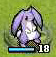## RubyQC

A conceptual QuickCheck library for Ruby

http://godfat.org/slide/2014-04-25-rubyqc/

## Who?godfat (Lin Jen-Shin)

• 2001~2004: C
• 2004~2009: ActionScript
• 2005~2008: C++
• 2006~2014: Ruby
• 2007~2014: (learning) Haskell

## Table of Contents

• Why Correctness

## Table of Contents

• Why Correctness
• Why QuickCheck
• RubyQC Examples
• Conclusion
In theoretical computer science, correctness of an algorithm is asserted when it is said that the algorithm is correct with respect to a specification.

## Project Management## Computers don't think for youUniverse

Program
Universe
Proofs
Program

## Table of Contents

• Why Correctness
• Why QuickCheck
• RubyQC Examples
• Conclusion

## +

``````
def plus x, y
x + y
end
``````
``````
def plus x, y
x + y
end
``````
What if we test all possible inputs?   Nah, it ain't gonna stop.
``````
-Infinity..+Infinity.each do |x|
-Infinity..+Infinity.each do |y|
end
end
``````
``````
def plus x, y
x + y
end
``````
What if we only sample it 100 times?
``````
100.times do
x, y = rand, rand

end
``````
``````
def plus x, y
x + y
end
``````
What if we only sample it 100 times?   Kinda work, but...
``````
100.times do
x, y = rand, rand
plus(x, y).should ==            #  ?
end
``````
``````
def plus x, y
x + y
end
``````
What if we only sample it 100 times?   Kinda work, but...
``````
100.times do
x, y = rand, rand
plus(x, y).should == x + y      #  ?
end
``````
``````
def plus x, y
x + y
end
``````
What if we only sample it 100 times?   Kinda work, but...
``````
100.times do
x, y = rand, rand
plus(x, y).should == plus(x, y) # ???
end
``````
``````
def plus x, y
x + y
end
``````
What if we only sample it 100 times?   Kinda work, but...
``````
100.times do
x, y = rand, rand
plus(x, y).should == plus(y, x) # ???
end
``````
``````

``````

``````

plus(x, y).should == plus(y, x) # ???

``````

## Commutativity

``````

x + y == y + x

``````

## Associativity

``````

(x + y) + z == x + (y + z)

``````

## Commutativity

``````
check(Fixnum, Fixnum) do |x, y|
plus(x, y).should == plus(y, x)
end
``````

## Associativity

``````

(x + y) + z == x + (y + z)

``````

## Commutativity

``````
check(Fixnum, Fixnum) do |x, y|
plus(x, y).should == plus(y, x)
end
``````

## Associativity

``````
check(Fixnum, Fixnum, Fixnum) do |x, y, z|
plus(plus(x, y), z).should == plus(x, plus(y, z))
end
``````

## Table of Contents

• Why Correctness
• Why QuickCheck
• RubyQC Examples
• Conclusion

## sort

``````
require 'bacon'
require 'rubyqc'

Bacon.summary_on_exit
Bacon::Context.include RubyQC::API

describe Array do
describe 'sort' do
should 'Any former should be <= any latter' do
check([Fixnum]*100).times(10) do |array|
array.sort.each_cons(2).each do |x, y|
x.should <= y
end
end
end
end
end
``````

## compare_by_identity

``````
describe 'Hash#compare_by_identity' do
should 'Treat diff str with the same contents diff when set' do
str = 'str'
forall([true, false],
[str, 'str'],
[str, 'nnf']) do |flag, a, b|
h = {}
h.compare_by_identity if flag
h[a] = h[b] = true

if (flag && a.object_id != b.object_id) ||
a != b
h.size.should == 2
else
h.size.should == 1
end
end
end
end
``````
``````
describe 'Hash#compare_by_identity' do
should 'Treat diff str with the same contents diff when set' do
str = 'str'
forall(booleans,
[str, 'str'],
[str, 'nnf']) do |flag, a, b|
h = {}
h.compare_by_identity if flag
h[a] = h[b] = true

if (flag && a.object_id != b.object_id) ||
a != b
h.size.should == 2
else
h.size.should == 1
end
end
end
end
``````
``````
describe 'Hash#compare_by_identity' do
should 'Treat diff arr with the same contents diff when set' do
arr =  
forall(booleans,
[arr,   ],
[arr,   ]) do |flag, a, b|
h = {}
h.compare_by_identity if flag
h[a] = h[b] = true

if (flag && a.object_id != b.object_id) ||
a != b
h.size.should == 2
else
h.size.should == 1
end
end
end
end
``````

## Custom Generator

``````
class User < Struct.new(:name, :gold)
def self.rubyqc
new(String.rubyqc, Fixnum.rubyqc)
end
end

describe User do
should 'Generate random users' do
check(User) do |user|
user     .should.kind_of User
user.name.should.kind_of String
user.gold.should.kind_of Fixnum
end
end
end
``````
``````
class User < Struct.new(:name, :gold)
def self.rubyqc
new(String.rubyqc, (0..99).rubyqc)
end

def give that, amount
if gold >= amount
self.gold -= amount
that.gold += amount
true
else
false
end
end
end
``````
``````
class User < Struct.new(:name, :gold)
def self.rubyqc
new(String.rubyqc, (0..99).rubyqc)
end
end

should 'Give gold to others' do
check(User, User, 0..99) do |a, b, gold|
total = a.gold + b.gold # Given
agold = a.gold

a.give(b, gold).should == gold <= agold # When

a.gold.should >= 0 # Then
b.gold.should >= 0
(a.gold + b.gold).should == total
end
end
``````

## Table of Contents

• Why Correctness
• Why QuickCheck
• RubyQC Examples
• Conclusion

## So with RubyQC

• We think properties (invariants) instead of scenarios

## Design of RubyQC

• Testing framework agnostic

## Q?

https://github.com/godfat/rubyqc

``````
gem install rubyqc``````